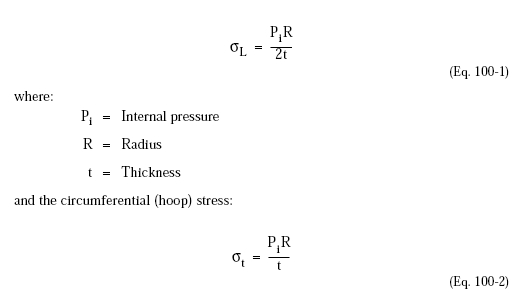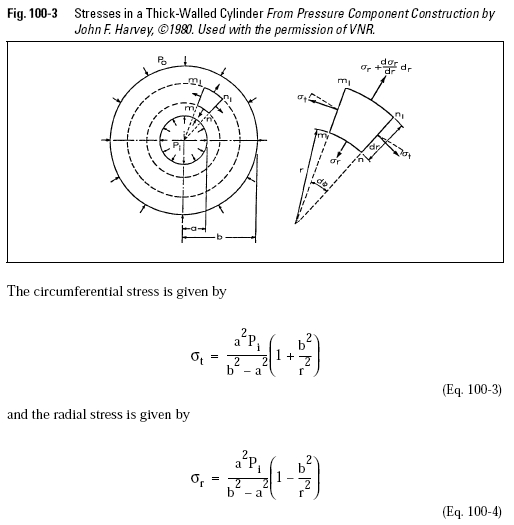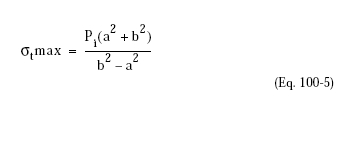﻿ Pressure Vessel Cylindrical Shells Under Internal Pressure – Oil & Gas Industry Technology Updates

## Pressure Vessel Cylindrical Shells Under Internal Pressure

The most important case in vessel design is a thin shell surface of revolution subjected to internal pressure. The internal pressure can be a uniform gas pressure or a liquid pressure varying along the axis of rotation due to the liquid head. In the latter case usually two calculations are performed to determine the stresses due to the equivalent gas pressure plus the stress in the lowest part of the shell due to the liquid weight.

Stresses in a closed-end-cylindrical shell under internal pressure Pi, computed from the conditions of static equilibrium (Figure 100-2) are the longitudinal (meridional) stress:As can be seen from these two equations, the hoop stress is always greater and determines the required thickness of the shell.

The equations above are accurate for thin wall cylinders (R/t > 10) under internal pressure. However, for thick wall cylinders (R/t < 10), the variation in stress from the inner to the outer surface becomes appreciable, and the above equations are not satisfactory.The Lamé, or thick-cylinder equations, are used to calculate the stresses (radial and circumferential) at any radius, r, in a thick wall cylinder as shown in Figure 100-3.where:
Pi = Internal pressure